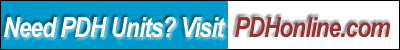Print this page

Basic Applied Finite Element Analysis

Robert B. Wilcox, P.E.

Course Outline

This course will cover the basics of linear static finite element analysis of a machine part to accurately determine stress distributions due to applied loads. It will focus on practical application and real-world issues related to finite element analysis, as opposed to theory. While just about any engineer can get a contour plot showing stresses, many do not understand the iterative nature of FEA and how to obtain meaningful results. This course will use a verifiable geometric example so the user can gain confidence in their ability to create and refine a mesh, loading, and boundary conditions which give accurate results. Students have the option to download a trial version of a popular FEA program and obtain hands-on experience of meshing, material property specification, load application, boundary condition application, solving, and interpretation of results. Symmetry modeling and convergence of results will also be covered.

• Prerequisites to Applying FEA
• Definitions
• Important Assumptions of Linear Static FEA
• Basic Analysis Types
• Model Symmetry
• Boundary Conditions
• Problem Solving Methodology
• Plane Stress Model Problem: Plate With a Hole
• Structured Vs. Unstructured Meshes
• Convergence
• Structural Model Problem: Planar Truss
• CAD Modeling Considerations for FEA
• Von Mises Stress
• 3-D Solid Model Example: Pressure Cylinder

The course includes a multiple-choice quiz at the end, which is designed to enhance the understanding of the course materials.

Learning Objective

At the conclusion of this course, the student will:

• Understand the basic assumptions of Linear Static Finite Element Analysis (FEA);
• Appreciate the prerequisite skills required to apply FEA methods;
• Understand basic FEA terminology;
• Learn some basic element types;
• Learn the difference between H-elements and P-elements;
• Be familiar with some of the limitations of Linear Static FEA;
• Comprehend considerations of the solid or planar CAD model relevant to the FEA model;
• Understand how to create a basic mesh;
• Be able to run a basic FEA analysis;
• Be familiar with mesh refinement;
• Understand plane stress models;
• Understand plane strain models;
• Have learned about symmetry models and boundary conditions;
• Understand axisymmetric models and boundary conditions;
• Be able to apply material properties to the model;
• Understand basic boundary conditions;
• Understand symmetry model boundary conditions;
• Understand application of loads to the model;
• Understand the concept of convergence;
• Understand element quality Issues;
• Know the difference between a structured and unstructured mesh;
• Interpret displacement and stress results;
• Understand the importance of manual checks on model results; and
• Understand von Mises Stress.

Intended Audience

This course is intended for mechanical and structural engineers and designers.

Benefit to Attendees

The attendee will obtain some experience-guided insight into modeling methods, pitfalls, and how to attain meaningful result from the FEA, particularly in the areas of mesh validity and convergence - two highly overlooked areas for newer users of FEA.

Course Introduction

Finite Element Analysis is a powerful tool for the mechanical or structural engineer, but it is easily misapplied, and results can easily by incorrect or misinterpreted by the uninitiated. Rather than focus on the mathematical theory behind FEA, this course will look at how to practically set up the model and get meaningful results. The focus will is on correct loads and boundary conditions, good meshes, convergence of results, and correct interpretation of results. The attendee will be downloading and installing a demo FEA program, and going through the steps of modeling using the program.

Course Content

The course content is in a PDF file (830 KB) Basic Applied Finite Element Analysis.

You may need to download the following FEM Demo Softwasre and sampel files:

Demo Software FEA Code.

Sampel Files

Course Summary

FEA can give good results and save much time and expense in development in testing provided that models are set up, run and interpreted correctly. A good understanding of model symmetry, constraints and loading, and mesh quality contribute to an accurate model. Through convergence, precision evaluation and element quality, the analyst can be confident of the accuracy of the results.